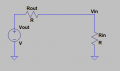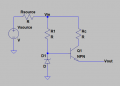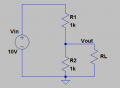# Output and Input Impedance

#### leo0001

Joined Sep 30, 2018
79
Hi,

I have severals questions :

1) The first one is what is the mathematical definition of input and output impedance :

According to me :
• Input impedance is equal to Zin = Vin/Iin
• Output impedance is equal to Zout = (Vout - Vin)/Iout, however I ve already seen that the output impedance is equal to Zout = Vout/Iout. If you can explain me how Zout can be equal to Vout/Iout, it will be very nice to you2)Here is a circuit, it is a voltage regulator with an emmiter follower :What is the output impedance of this circuit ?

Can you please detail your calcul ? I m not interested in the result, i m interested in how you proceed to compute the output impedance.

Thank you very much !

Have a nice day#### crutschow

Joined Mar 14, 2008
31,135
The input impedance is ΔVin/ΔIn.
The output impedance is ΔVout/ΔIout.
Δ is the change in that value.

So for the emitter follower, you measure the output voltage and current for a given load.
You then vary the load and again measure the output voltage and current.
The output impedance is then (V1-V2)/(I1-I2).
For an emitter follower this impedance will be fairly low.

•leo0001

#### leo0001

Joined Sep 30, 2018
79
I do not understandSuppose I am in DC and there is no variation on the load, the output impedance is equal to 0 ? I do not understand how can we consider that the output impedance is equal to the ratio between the variation of Vout and the variation of Vin. Please tell meI could understand this definition is correct if we do a small signal analysis.

#### crutschow

Joined Mar 14, 2008
31,135
I do not understand how can we consider that the output impedance is equal to the ratio between the variation of Vout and the variation of Vin.
That definition is not correct.
The output impedance is not related to Vin.

#### leo0001

Joined Sep 30, 2018
79

#### MisterBill2

Joined Jan 23, 2018
13,809
In a regulated power supply the effective output impedance is very low, because changes in the load current do not produce changes in the output voltage, or at least not much change in the output voltage. So that is the effective output impedance, And if there is no output current, then the output impedance does not matter..

•leo0001

#### leo0001

Joined Sep 30, 2018
79
Thanks for your reply. I do not agree with you. If the regulated power supply is correctly design, the effective output impedance is very low. I do not think actually that the circuit I show you is the best for this (at least it is possible to do better) and that's why I will be happy to know how can I proceed to compute the output impedance of that circuit.

#### Bordodynov

Joined May 20, 2015
3,025

#### Attachments

• 2.3 KB Views: 2
•leo0001

#### MisterBill2

Joined Jan 23, 2018
13,809
The effective output impedance can be calculated by using the change in output voltage with the change in output current. If the output voltage drops one volt with an increase of one amp then the effective internal resistance is one ohm. Of course, since the active elements are not linear, that output effective resistance may be different at different load currents.
And if there is no output current, resulting in no change in voltage, then no, it does not matter. Any changes in output voltage not caused by output current are from other sources, such as temperature change or input voltage change.
And certainly there are many regulator circuits better than the elementary one posted.

•leo0001

#### Jony130

Joined Feb 17, 2009
5,435
1) The first one is what is the mathematical definition of input and output impedance :
I will give you an example of how you can find the output impedance.
And the circuit will look like this:And from the Zout definition we know that :

Zout = ΔVout/ΔIout

So in our circuit we have :

Vout_1 = 5V and Iout_1 = 0A for RL = ∞;

And now If we add RL = 500Ω the output voltage becomes Vout_2 = 5V* 0.5kΩ/(0.5kΩ + 0.5kΩ) = 2.5V and Iout_2 = 5V/(0.5kΩ + 0.5kΩ) = 5mA

Therefore Zout = (5V - 2.5V)/(5mA - 0A) = 500Ω

As you can see we can measure the output impedance or calculate it as I did with the help of a Thevenin's theorem.
http://www.zen22142.zen.co.uk/Theory/inzoz.htm

•Thank you for all your reply. I appreciate it